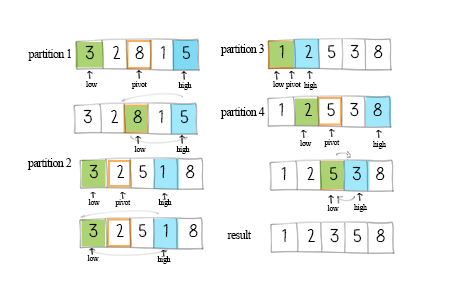Quicksort partitions the array into two subarrays. This is done by calling a partition method. In this method, a pivot is the key value to determine when to swap unordered elements. It returns the index of the partition. Then the sorting method calls itself to sort these two subarrays.In the partition method, there are different ways to define pivot. For example, it can be the first, last or the middle item in the subarray. In this solution, the pivot is the middle of the array. The formula of its index is mid=low+(high-low)/2.

Meanwhile in the partition method, there are two pointers, low points to the first element. high points to the last element. Increase the low pointer if the values it points are less than the pivot. Decrease the high pointer if the values are larger than the pivot. After moving the pointers, if the low pointer is still less equal than the high pointer, swap the elements in the array. After each partition, either the largest element swaps to the right, or the smallest element swaps to the left.

Quicksort uses divide and conquer technique as merge sort. It operates in O(nlogn) time. It is faster than simple sorting such as bubble sort, selection sort and insertion sort.

## Doodle